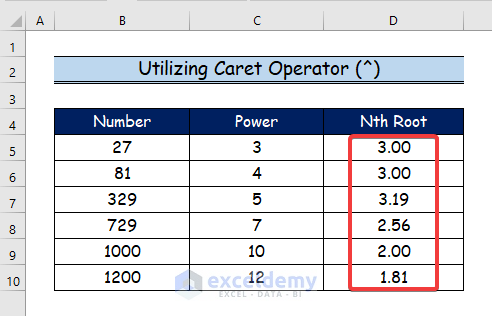# How to Find Nth Root in Excel (2 Easy Ways)

Get FREE Advanced Excel Exercises with Solutions!

The letter n stands for any number of roots for a particular number (i.e. 1,2,3, etc.). To discover the nth root of a number, we must first identify the number whose multiplication by “n” yields the number for which the nth root must be found. As a result, the provided integer’s nth root is the number that is multiplied by itself n times. In this article, you will learn to find the nth root in Excel.

In the following two steps, we will find the nth root in Excel by applying the Power function and utilizing the Caret (^) operator. Let’s suppose we have a sample data set.## 1. Using Power Function to Find Nth Root in Excel

The built-in POWER function in Excel determines the power of any number. This function requires two arguments; number and power. Power is the exponent by which the base number is raised, and the number is the base number. In the first step, you will learn to find the nth root in Excel. The general syntax of the POWER function is shown below.

`=Power (number, power)`

Argument

• Number – This argument represents the base number we want to raise to a certain power.
• Power – This argument represents the exponent to which the base number is raised.

Step 1:

• Firstly, select cell D5.
• Then, type the following formula.
`=POWER(B5,1/C5)`
• Here, the B5 cell conveys the power argument.
• And, the C5 cell represents the exponent argument.Step 2:

• Now press ENTER, and the result will show the nth root of the first number in the D5 cell.
• Then, use the Fill Handle tool and drag it down from cell D5 to D10.Step 3:

• Finally, we will get the nth roots of all the numbers in column D.Read More: How to Calculate Square Root in Excel

## 2. Applying Caret Operator (^) to Find Nth Root

Mathematical equations frequently use the exponential operator, which is available in Excel. The caret (^) operator represents power in Excel. While working on various Excel calculations, we employ this caret operator in less complex expressions and calculations. Here, we’ll use Excel’s caret operator to determine the nth root.

Step 1:

• Firstly, select cell D5.
• Then, type the following formula.
`=B5^(1/C5)`Step 2:

• When you press ENTER, cell D5 will display the nth root of the initial number.
• Next, move the Fill Handle tool from cell D5 to D10 while using the arrow keys.Step 3:

• Lastly, you will see all the nth roots of all the numbers in the D column here.

##You may download the following Excel workbook for better understanding and practice it by yourself.

## Conclusion

In this article, we’ve covered 2 handy methods to find the nth root in Excel. We sincerely hope you enjoyed and learned a lot from this article. If you have any questions, comments, or recommendations, kindly leave them in the comment section below.

## What is ExcelDemy?

ExcelDemy Learn Excel & Excel Solutions Center provides free Excel tutorials, free support , online Excel training and Excel consultancy services for Excel professionals and businesses. Feel free to contact us with your Excel problems.Bishawajit Chakraborty

I'm Bishawajit Chakraborty. Hello. I graduated from Rajshahi University of Engineering & Technology (RUET) with a degree in Mechanical Engineering. I'm working with ExcelDemy as a Content Developer for Excel & VBA. You can visit our website, Exceldemy if you'd like to read my published articles on MS Excel and VBA.

We will be happy to hear your thoughtsAdvanced Excel Exercises with Solutions PDF